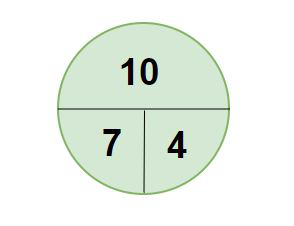Open in App
Not now

# Expected Value Formula

• Last Updated : 02 Jun, 2022

Probability is a proportion of the likelihood of an event occurring. It is a measure of the chance of happening or non-happening of the outcomes of a random experiment. The Expected values represent what we expect the outcome to be if we run an experiment many times. It is basically the product of the value of each outcome and its probability. Let’s take a look at the formula for expected value,

### Expected Value formula

The general formula to find the Expected value for multiple events is,

E(X) = Σ P(X) × X

Where,

E(X) – the expected value

P(X) – the probability of the event

X – the event.

Utilizations of Expected Value Formula

Expected value is characterized as the result of the probability and the value of every result.

1. It gives a fast knowledge into the way of behaving of any irregular variable.
2. Ordinarily utilized in finance as it shows the anticipated value of future ventures.
3. It is utilized for decision-making in different fields.

### Sample Questions

Question 1: Using the expected value formula, find the expected value for the following probability distribution.

Solution:

Using Expected Value for multiple events,

Formula: E(X) = Σ P(X) × X

E(X) = 1 × 0.25 + 2 × 1.35 + 3 × 0.45 + 4 × 0.11

= 4.74

Therefore, the Expected Value of the given probability distribution is 4.74

Question 2: Hari plays a game in which there are only two outcomes. The cost to play the game is 5000. if he wins, he receives 15000. the probability of winning is 30%. what is the expected value for winning a single game on average?

Solution:

Using Expected Value Formula,

E(X) = Σ P(X) × X

= 15000(0.30) + (-5000)(0.70)

= 4500 – 3500

= 1000

The Expected Value for winning a single game on average is 1000.

Question 3: A company generates a profit of 4000 for each computer they sell. The company loses 15000 for every computer that is returned due to some defect. If 3 out of every 150 computers that they produce is defective, what is the expected value of profit per computer?

Solution:

Using Expected Value Formula,

E(X) = Σ P(X) × X

= 4000(0.97) + (-15000)(0.03)

= 3880 – 450

= 3430

The Expected Value of profit per computer is 3430.

Question 4: Raffle tickets are being sold for 200 rupees. There are 4 prizes being given out. They are 500, 1000, 800, and 650. If 2000 tickets are sold, find the expected gain?

Solution:

Using Expected Value Formula,

E(X) = Σ P(X) × X

= 300(1/2000) + 800(1/2000) + 600(1/2000) + 450(1/2000) + (-200)(1996/2000)

= (300 + 800 + 600 + 450)/2000 + (-200)(1996/2000)

= 2150/2000 + (-200)(1996/2000)

= 1.075 + (-200)(1996/2000)

= -198.525

There is an Expected Gain of -198.525 (Or) An expected Loss of 198.525.

Question 5: There is a game that you are considering playing. You pay \$1 for a ticket to roll a 6-sided die. If you roll a 1, 2, 4,  or 6, you lose. If you roll a 3, you get your money back. If you roll a 5, you win \$4. What is the expected value of playing this game and what is a fair price for this game?

Solution:

Using Expected Value Formula,

E(X) = Σ P(X) × X

= (-1)(1/6) +  (-1)(1/6) + (0)(1/6) + (-1)(1/6) + (3)(1/6) +  (-1)(1/6)

= (-1-1-1+3-1)/6

= -1/6

= -0.166

Fair Price =  – 0.166

= 0.83

Fair price = current cost to play + expected value

Question 6: A venue expects 5000 people to attend if it does not rain, and 1000 people to attend if it does rain. The chance of rain is 70%. What is the expected attendance?

Solution:

Using Expected Value Formula,

E(X) = Σ P(X) × X

= 1000(0.7) + 5000(0.3)

= 2200

The expected attendance is 2200.

Question 7: A person spins the wheel and is rewarded the amount indicated by the pointer. What is the expected value of a spin?Solution:

Using Expected Value Formula,

E(X) = Σ P(X) × X

= 10(1/2) + 7(1/4) +4(1/4)

= 5 + 1.75 + 1

= 7.75

Therefore, the expected value of a spin is 7.75.

My Personal Notes arrow_drop_up
Related Articles